The OpenFOAM Foundation
icoPolynomial< Specie, PolySize > Class Template Reference

Incompressible, polynomial form of equation of state, using a polynomial function for density. More...

Inheritance diagram for icoPolynomial< Specie, PolySize >: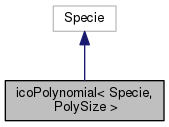[legend]
Collaboration diagram for icoPolynomial< Specie, PolySize >:[legend]

## Public Member Functions

icoPolynomial (const Specie &sp, const Polynomial< PolySize > &rhoPoly)
Construct from components. More...

icoPolynomial (const dictionary &dict)
Construct from dictionary. More...

icoPolynomial (const word &name, const icoPolynomial &)
Construct as named copy. More...

autoPtr< icoPolynomialclone () const
Construct and return a clone. More...

scalar rho (scalar p, scalar T) const
Return density [kg/m^3]. More...

scalar H (const scalar p, const scalar T) const
Return enthalpy departure [J/kg]. More...

scalar Cp (scalar p, scalar T) const
Return Cp departure [J/(kg K]. More...

scalar S (const scalar p, const scalar T) const
Return entropy [J/(kg K)]. More...

scalar psi (scalar p, scalar T) const
Return compressibility rho/p [s^2/m^2]. More...

scalar Z (scalar p, scalar T) const
Return compression factor []. More...

scalar CpMCv (scalar p, scalar T) const
Return (Cp - Cv) [J/(kg K]. More...

void write (Ostream &os) const
Write to Ostream. More...

void operator= (const icoPolynomial &)

void operator+= (const icoPolynomial &)

void operator*= (const scalar)

## Static Public Member Functions

static autoPtr< icoPolynomialNew (const dictionary &dict)

static word typeName ()
Return the instantiated type name. More...

## Static Public Attributes

static const bool incompressible = true
Is the equation of state is incompressible i.e. rho != f(p) More...

static const bool isochoric = false
Is the equation of state is isochoric i.e. rho = const. More...

## Friends

icoPolynomial operator+ (const icoPolynomial &, const icoPolynomial &)

icoPolynomial operator* (const scalar s, const icoPolynomial &)

icoPolynomial operator== (const icoPolynomial &, const icoPolynomial &)

Ostreamoperator (Ostream &, const icoPolynomial &)

## Detailed Description

### template<class Specie, int PolySize = 8> class Foam::icoPolynomial< Specie, PolySize >

Incompressible, polynomial form of equation of state, using a polynomial function for density.

Usage
Property Description
rhoCoeffs<8> Density polynomial coefficients

Example of the specification of the equation of state:

    equationOfState
{
rhoCoeffs<8>    ( 1000 -0.05 0.003 0 0 0 0 0 );
}

The polynomial expression is evaluated as so: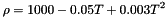Note
Input in [kg/m3], but internally uses [kg/m3/kmol].
Source files
Foam::Polynomial

Definition at line 82 of file icoPolynomial.H.

## ◆ icoPolynomial() [1/3]

 icoPolynomial ( const Specie & sp, const Polynomial< PolySize > & rhoPoly )
inline

Construct from components.

Definition at line 32 of file icoPolynomialI.H.

References Foam::name().

Here is the call graph for this function: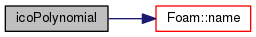## ◆ icoPolynomial() [2/3]

 icoPolynomial ( const dictionary & dict )

Construct from dictionary.

Definition at line 37 of file icoPolynomial.C.

## ◆ icoPolynomial() [3/3]

 icoPolynomial ( const word & name, const icoPolynomial< Specie, PolySize > & )
inline

Construct as named copy.

## ◆ clone()

 Foam::autoPtr< Foam::icoPolynomial< Specie, PolySize > > clone ( ) const
inline

Construct and return a clone.

Definition at line 58 of file icoPolynomialI.H.

## ◆ New()

 Foam::autoPtr< Foam::icoPolynomial< Specie, PolySize > > New ( const dictionary & dict )
inlinestatic

Definition at line 69 of file icoPolynomialI.H.

References dict, and icoPolynomial< Specie, PolySize >::rho().

Here is the call graph for this function: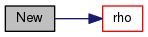## ◆ typeName()

 static word typeName ( )
inlinestatic

Return the instantiated type name.

Definition at line 155 of file icoPolynomial.H.

## ◆ rho()

 Foam::scalar rho ( scalar p, scalar T ) const
inline

Return density [kg/m^3].

Definition at line 82 of file icoPolynomialI.H.

References icoPolynomial< Specie, PolySize >::H().

Referenced by icoPolynomial< Specie, PolySize >::New().

Here is the call graph for this function: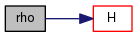Here is the caller graph for this function: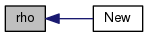## ◆ H()

 Foam::scalar H ( const scalar p, const scalar T ) const
inline

Return enthalpy departure [J/kg].

Definition at line 93 of file icoPolynomialI.H.

References icoPolynomial< Specie, PolySize >::Cp().

Referenced by icoPolynomial< Specie, PolySize >::rho().

Here is the call graph for this function: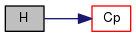Here is the caller graph for this function: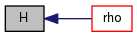## ◆ Cp()

 Foam::scalar Cp ( scalar p, scalar T ) const
inline

Return Cp departure [J/(kg K].

Definition at line 104 of file icoPolynomialI.H.

References icoPolynomial< Specie, PolySize >::S().

Referenced by icoPolynomial< Specie, PolySize >::H().

Here is the call graph for this function: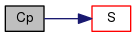Here is the caller graph for this function: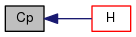## ◆ S()

 Foam::scalar S ( const scalar p, const scalar T ) const
inline

Return entropy [J/(kg K)].

Definition at line 115 of file icoPolynomialI.H.

References icoPolynomial< Specie, PolySize >::psi().

Referenced by icoPolynomial< Specie, PolySize >::Cp().

Here is the call graph for this function: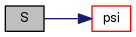Here is the caller graph for this function: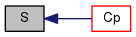## ◆ psi()

 Foam::scalar psi ( scalar p, scalar T ) const
inline

Return compressibility rho/p [s^2/m^2].

Definition at line 126 of file icoPolynomialI.H.

References icoPolynomial< Specie, PolySize >::Z().

Referenced by icoPolynomial< Specie, PolySize >::S().

Here is the call graph for this function: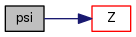Here is the caller graph for this function: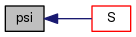## ◆ Z()

 Foam::scalar Z ( scalar p, scalar T ) const
inline

Return compression factor [].

Definition at line 137 of file icoPolynomialI.H.

References icoPolynomial< Specie, PolySize >::CpMCv().

Referenced by icoPolynomial< Specie, PolySize >::psi().

Here is the call graph for this function: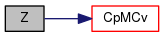Here is the caller graph for this function: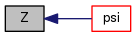## ◆ CpMCv()

 Foam::scalar CpMCv ( scalar p, scalar T ) const
inline

Return (Cp - Cv) [J/(kg K].

Definition at line 148 of file icoPolynomialI.H.

References Foam::sqr().

Referenced by icoPolynomial< Specie, PolySize >::Z().

Here is the call graph for this function: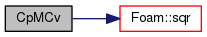Here is the caller graph for this function: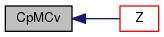## ◆ write()

 void write ( Ostream & os ) const

Write to Ostream.

Definition at line 53 of file icoPolynomial.C.

Here is the call graph for this function: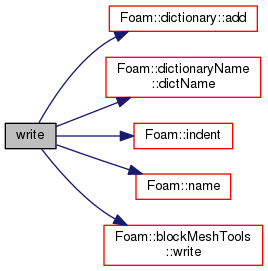## ◆ operator=()

 void operator= ( const icoPolynomial< Specie, PolySize > & )
inline

Definition at line 161 of file icoPolynomialI.H.

## ◆ operator+=()

 void operator+= ( const icoPolynomial< Specie, PolySize > & )
inline

Definition at line 173 of file icoPolynomialI.H.

References Foam::mag(), Y, Y1, and Y2.

Here is the call graph for this function: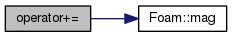## ◆ operator*=()

 void operator*= ( const scalar s )
inline

Definition at line 191 of file icoPolynomialI.H.

References Foam::mag(), s(), Y1, and Y2.

Here is the call graph for this function: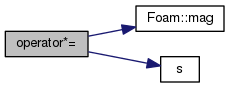## ◆ operator+

 icoPolynomial operator+ ( const icoPolynomial< Specie, PolySize > & , const icoPolynomial< Specie, PolySize > & )
friend

## ◆ operator*

 icoPolynomial operator* ( const scalar s, const icoPolynomial< Specie, PolySize > & )
friend

## ◆ operator==

 icoPolynomial operator== ( const icoPolynomial< Specie, PolySize > & , const icoPolynomial< Specie, PolySize > & )
friend

## ◆ operator

 Ostream& operator ( Ostream & , const icoPolynomial< Specie, PolySize > & )
friend

## ◆ incompressible

 const bool incompressible = true
static

Is the equation of state is incompressible i.e. rho != f(p)

Definition at line 164 of file icoPolynomial.H.

## ◆ isochoric

 const bool isochoric = false
static

Is the equation of state is isochoric i.e. rho = const.

Definition at line 167 of file icoPolynomial.H.

The documentation for this class was generated from the following files:
• src/thermophysicalModels/specie/equationOfState/icoPolynomial/icoPolynomial.H
• src/thermophysicalModels/specie/equationOfState/icoPolynomial/icoPolynomial.C
• src/thermophysicalModels/specie/equationOfState/icoPolynomial/icoPolynomialI.H